# 1. 社团划分

## 0x1：社区是什么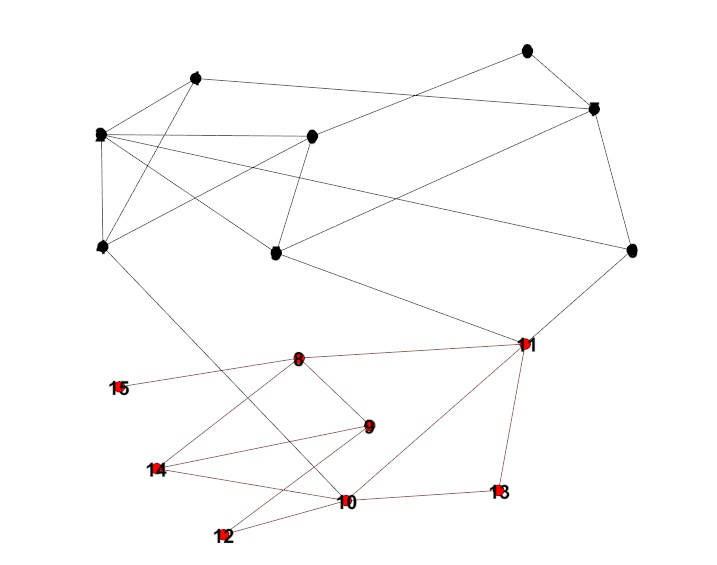## 0x2：社区划分的出发点和意图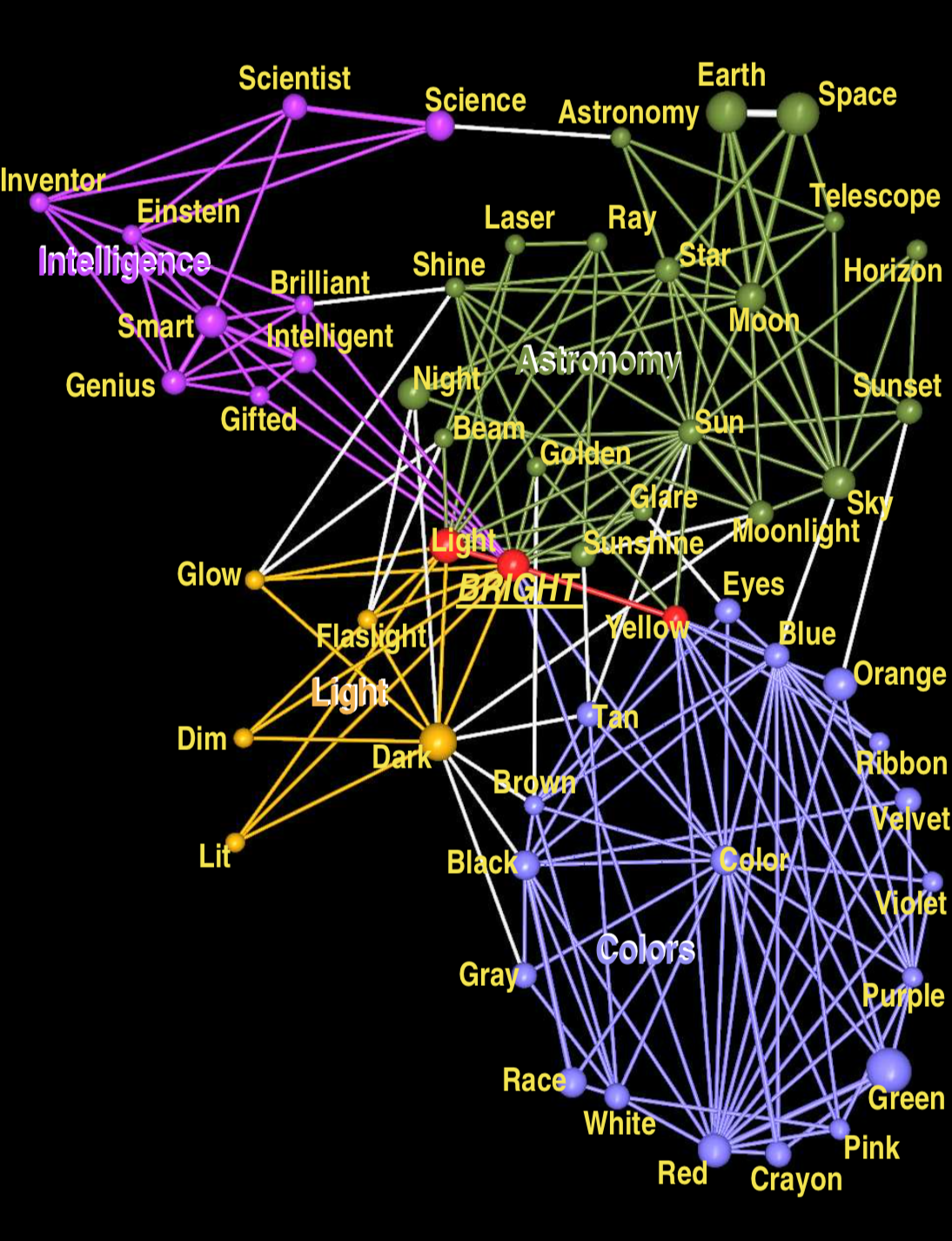## 0x3：节点间存在连接的抽象本质 - 逻辑拓朴结构

1. 节点代表消费者：节点间的连接代表了它们共同购买了一批书籍，weight代表共同购买的书籍数；
2. 节点代表DNS域名：节点间的连接代表了它们拥有一批共同的src client ip（客户端），weight代表了共同的src_ip数量；

## 0x4：什么时候可以使用社区发现算法

(Newman and Gievan 2004) A community is a subgraph containing nodes which are more densely linked to each other than to the rest of the graph or equivalently, a graph has a community structure if the number of links into any subgraph is higher than the number of links between those subgraphs.

## 0x5：社区划分的思路概要

https://stackoverflow.com/questions/21814235/how-can-modularity-help-in-network-analysis
http://iopscience.iop.org/article/10.1088/1742-5468/2008/10/P10008/fulltext/
https://www.researchgate.net/publication/1913681_Fast_Unfolding_of_Communities_in_Large_Networks?enrichId=rgreq-d403e26a5cb211b7053c36946c71acb3-XXX&enrichSource=Y292ZXJQYWdlOzE5MTM2ODE7QVM6MTAxOTUyNjc5NTc5NjY3QDE0MDEzMTg4MjE3ODA%3D&el=1_x_3&_esc=publicationCoverPdf
https://www.jianshu.com/p/4ebe42dfa8ec
https://blog.csdn.net/u011089523/article/details/79090453
《Fast Unfolding of Communities in Large Networks》

# 2. LOUVAIN算法模型

Louvain算法是一种基于多层次（逐轮启发式迭代）优化Modularity的算法。Modularity函数最初被用于衡量社区发现算法结果的质量，它能够刻画发现的社区的紧密程度。

## 0x1：Modularity的定义 - 描述社区内紧密程度的值Q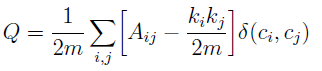A为邻接矩阵，Aij代表了节点 i 和节点 j 之间 边的权重，网络不是带权图时，所有边的权重可以看做是 1；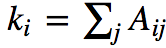是所有与节点 i 相连的 边的权重之和（度数），kj也是同样；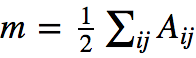表示所有边的权重之和（边的数目），充当归一化的作用；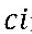是节点 i 的社区，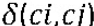函数表示若节点 i 和节点 j 在同一个社区内，则返回 1，否则返回 0；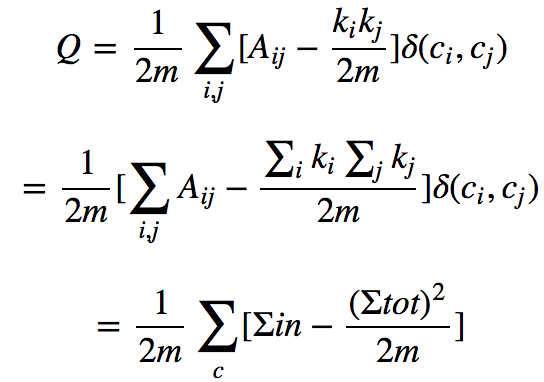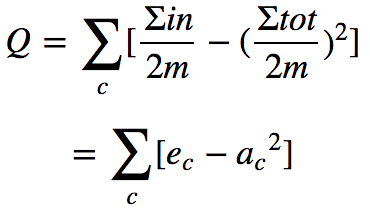modularity Q的计算公式背后体现了这种思想：社区内部边的权重减去所有与社区节点相连的边的权重和，对无向图更好理解，即社区内部边的度数减去社区内节点的总度数。

## 0x2：模块度增量 delta Q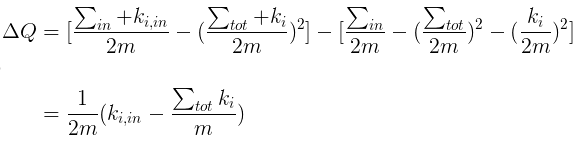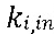代表由节点 i 入射集群 C 的权重之和；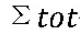代表入射集群 C 的总权重；ki 代表入射节点 i 的总权重；

# 3. LOUVAIN算法策略

Louvain算法是基于模块度的社区发现算法，该算法在效率和效果上都表现较好，并且能够发现层次性的社区结构，其优化目标是：最大化整个社区网络的模块度。

## 0x1：算法思想的联想

Louvain算法包括两个阶段，在步骤一它不断地遍历网络中的结点，尝试将单个结点加入能够使modularity提升最大的社区中，直到所有结点都不再变化。在步骤二，它处理第一阶段的结果，将一个个小的社区归并为一个超结点来重新构造网络，这时边的权重为两个结点内所有原始结点的边权重之和。迭代这两个步骤直至算法稳定。

1. 两台主机拥有类似的网络对外发包模式
2. 两台主机间拥有累计的event log序列
4. 在netword gateway上发现了类似的网络raw流量，也可以反过来用一直的label流量特征进行有监督的聚类
..

## 0x2：关于启发式/贪婪思想的社区发现的进一步思考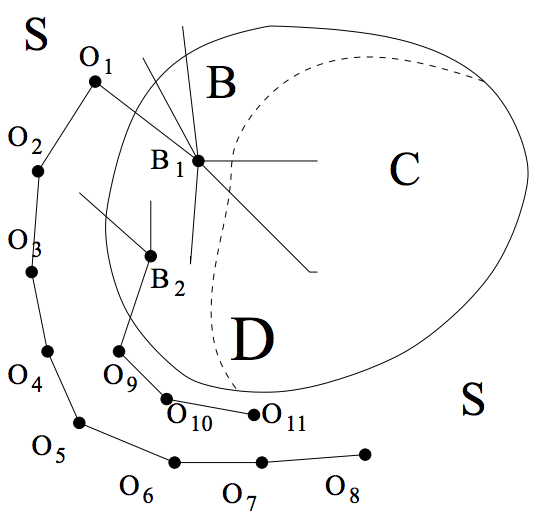## 0x3：社区发现（聚类）的效果非常依赖于weight权重计算的策略和方法

louvain社区发现是将一个无向权重图，转化为多个节点集合，每个节点集合代表了一个社区。

A - B：2
A - C：2
B - C：2
D - E：12（明显和2不一样）
D - F：13
E - F：14
F - G：11
A - D：1（社区间存在弱关系）
B - F：1

A/B/C、D/E/F

# 4. LOUVAIN算法流程

## 0x2：算法时间复杂度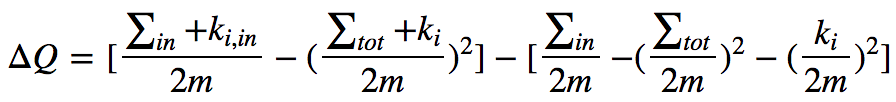DeltaQ 分了两部分，前面部分表示把节点i加入到社区c后的模块度，后一部分是节点i作为一个独立社区和社区c的模块度

https://blog.csdn.net/xuanyuansen/article/details/68941507
https://www.cnblogs.com/fengfenggirl/p/louvain.html
http://www.cnblogs.com/allanspark/p/4197980.html
https://github.com/gephi/gephi/wiki
https://blog.csdn.net/qq547276542/article/details/70175157

# 5. A Python implementation of the Louvain method to find communities in large networks

#!/usr/bin/env python3
# -*- coding: utf-8 -*-

'''
Implements the Louvain method.
Input: a weighted undirected graph
Ouput: a (partition, modularity) pair where modularity is maximum
'''
class PyLouvain:

'''
Builds a graph from _path.
_path: a path to a file containing "node_from node_to" edges (one per line)
'''
@classmethod
def from_file(cls, path):
f = open(path, 'r')
f.close()
nodes = {}
edges = []
for line in lines:
n = line.split()
if not n:
break
nodes[n] = 1
nodes[n] = 1
w = 1
if len(n) == 3:
w = int(n)
edges.append(((n, n), w))
# rebuild graph with successive identifiers
nodes_, edges_ = in_order(nodes, edges)
print("%d nodes, %d edges" % (len(nodes_), len(edges_)))
return cls(nodes_, edges_)

'''
Builds a graph from _path.
_path: a path to a file following the Graph Modeling Language specification
'''
@classmethod
def from_gml_file(cls, path):
f = open(path, 'r')
f.close()
nodes = {}
edges = []
current_edge = (-1, -1, 1)
in_edge = 0
for line in lines:
words = line.split()
if not words:
break
if words == 'id':
nodes[int(words)] = 1
elif words == 'source':
in_edge = 1
current_edge = (int(words), current_edge, current_edge)
elif words == 'target' and in_edge:
current_edge = (current_edge, int(words), current_edge)
elif words == 'value' and in_edge:
current_edge = (current_edge, current_edge, int(words))
elif words == ']' and in_edge:
edges.append(((current_edge, current_edge), 1))
current_edge = (-1, -1, 1)
in_edge = 0
nodes, edges = in_order(nodes, edges)
print("%d nodes, %d edges" % (len(nodes), len(edges)))
return cls(nodes, edges)

'''
Initializes the method.
_nodes: a list of ints
_edges: a list of ((int, int), weight) pairs
'''
def __init__(self, nodes, edges):
self.nodes = nodes
self.edges = edges
# precompute m (sum of the weights of all links in network)
#            k_i (sum of the weights of the links incident to node i)
self.m = 0
self.k_i = [0 for n in nodes]
self.edges_of_node = {}
self.w = [0 for n in nodes]

for e in edges:
self.m += e
self.k_i[e] += e
self.k_i[e] += e # there's no self-loop initially
# save edges by node
if e not in self.edges_of_node:
self.edges_of_node[e] = [e]
else:
self.edges_of_node[e].append(e)
if e not in self.edges_of_node:
self.edges_of_node[e] = [e]
elif e != e:
self.edges_of_node[e].append(e)
# access community of a node in O(1) time
self.communities = [n for n in nodes]
self.actual_partition = []

'''
Applies the Louvain method.
'''
def apply_method(self):
network = (self.nodes, self.edges)
best_partition = [[node] for node in network]
best_q = -1
i = 1
while 1:
i += 1
partition = self.first_phase(network)
q = self.compute_modularity(partition)
partition = [c for c in partition if c]
# clustering initial nodes with partition
if self.actual_partition:
actual = []
for p in partition:
part = []
for n in p:
part.extend(self.actual_partition[n])
actual.append(part)
self.actual_partition = actual
else:
self.actual_partition = partition
if q == best_q: # 如果本轮迭代modularity没有改变，则认为收敛，停止
break
network = self.second_phase(network, partition)
best_partition = partition
best_q = q
return (self.actual_partition, best_q)

'''
Computes the modularity of the current network.
_partition: a list of lists of nodes
'''
def compute_modularity(self, partition):
q = 0
m2 = self.m * 2
for i in range(len(partition)):
q += self.s_in[i] / m2 - (self.s_tot[i] / m2) ** 2
return q

'''
Computes the modularity gain of having node in community _c.
_node: an int
_c: an int
_k_i_in: the sum of the weights of the links from _node to nodes in _c
'''
def compute_modularity_gain(self, node, c, k_i_in):
return 2 * k_i_in - self.s_tot[c] * self.k_i[node] / self.m

'''
Performs the first phase of the method.
_network: a (nodes, edges) pair
'''
def first_phase(self, network):
# make initial partition
best_partition = self.make_initial_partition(network)
while 1:
improvement = 0
for node in network:
node_community = self.communities[node]
# default best community is its own
best_community = node_community
best_gain = 0
# remove _node from its community
best_partition[node_community].remove(node)
for e in self.edges_of_node[node]:
if e == e:
continue
if e == node and self.communities[e] == node_community or e == node and self.communities[e] == node_community:
self.s_in[node_community] -= 2 * (best_shared_links + self.w[node])
self.s_tot[node_community] -= self.k_i[node]
self.communities[node] = -1
communities = {} # only consider neighbors of different communities
for neighbor in self.get_neighbors(node):
community = self.communities[neighbor]
if community in communities:
continue
communities[community] = 1
for e in self.edges_of_node[node]:
if e == e:
continue
if e == node and self.communities[e] == community or e == node and self.communities[e] == community:
# compute modularity gain obtained by moving _node to the community of _neighbor
if gain > best_gain:
best_community = community
best_gain = gain
# insert _node into the community maximizing the modularity gain
best_partition[best_community].append(node)
self.communities[node] = best_community
self.s_in[best_community] += 2 * (best_shared_links + self.w[node])
self.s_tot[best_community] += self.k_i[node]
if node_community != best_community:
improvement = 1
if not improvement:
break
return best_partition

'''
Yields the nodes adjacent to _node.
_node: an int
'''
def get_neighbors(self, node):
for e in self.edges_of_node[node]:
if e == e: # a node is not neighbor with itself
continue
if e == node:
yield e
if e == node:
yield e

'''
Builds the initial partition from _network.
_network: a (nodes, edges) pair
'''
def make_initial_partition(self, network):
partition = [[node] for node in network]
self.s_in = [0 for node in network]
self.s_tot = [self.k_i[node] for node in network]
for e in network:
if e == e: # only self-loops
self.s_in[e] += e
self.s_in[e] += e
return partition

'''
Performs the second phase of the method.
_network: a (nodes, edges) pair
_partition: a list of lists of nodes
'''
def second_phase(self, network, partition):
nodes_ = [i for i in range(len(partition))]
# relabelling communities
communities_ = []
d = {}
i = 0
for community in self.communities:
if community in d:
communities_.append(d[community])
else:
d[community] = i
communities_.append(i)
i += 1
self.communities = communities_
# building relabelled edges
edges_ = {}
for e in network:
ci = self.communities[e]
cj = self.communities[e]
try:
edges_[(ci, cj)] += e
except KeyError:
edges_[(ci, cj)] = e
edges_ = [(k, v) for k, v in edges_.items()]
# recomputing k_i vector and storing edges by node
self.k_i = [0 for n in nodes_]
self.edges_of_node = {}
self.w = [0 for n in nodes_]
for e in edges_:
self.k_i[e] += e
self.k_i[e] += e
if e == e:
self.w[e] += e
if e not in self.edges_of_node:
self.edges_of_node[e] = [e]
else:
self.edges_of_node[e].append(e)
if e not in self.edges_of_node:
self.edges_of_node[e] = [e]
elif e != e:
self.edges_of_node[e].append(e)
# resetting communities
self.communities = [n for n in nodes_]
return (nodes_, edges_)

'''
Rebuilds a graph with successive nodes' ids.
_nodes: a dict of int
_edges: a list of ((int, int), weight) pairs
'''
def in_order(nodes, edges):
# rebuild graph with successive identifiers
nodes = list(nodes.keys())
nodes.sort()
i = 0
nodes_ = []
d = {}
for n in nodes:
nodes_.append(i)
d[n] = i
i += 1
edges_ = []
for e in edges:
edges_.append(((d[e], d[e]), e))
return (nodes_, edges_)

node
[
id 16
label "Betrayal"
value "c"
]
node
[
id 17
label "Shut Up and Sing"
value "c"
]
node
[
id 18
label "Meant To Be"
value "n"
]
node
[
id 19
label "The Right Man"
value "c"
]http://www.cnblogs.com/allanspark/p/4197980.html
https://arxiv.org/pdf/0803.0476.pdf
https://github.com/LittleHann/pylouvain
http://www.cnblogs.com/allanspark/p/4197980.html
https://www.jianshu.com/p/e543dc63454f

# 6. 其他社区发现算法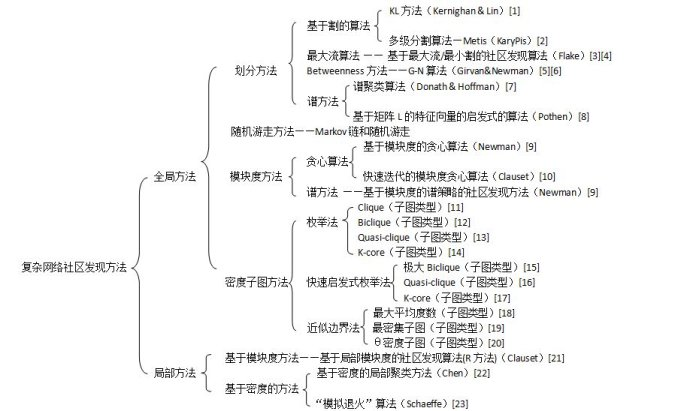http://blog.sina.com.cn/s/blog_63891e610101722t.html
https://wenku.baidu.com/view/36fa145a3169a4517623a313.html